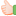• # Thread: Nested If/Else statements

1. ## Nested If/Else statements

Hi there,

I wonder if anyone can help nesting several If/Else statements in VW...

ThanksReply With Quote

2. Hi, please explain what you need to do.

VaultWiki's IF condition can only test 1 expression at a time (such as A == B), or a not-empty on a list of parameters (A B C D) which is true if any of A,B,C,D has content. So for more complicated expressions, like a logical AND, you would need to use nested IF statements.

You can simplify the IF statements around content and avoid confusion by making new local variables.

Code:
```[if="{{{x}}}==1"][if="{{{y}}}==2"]
[var]set|X1_and_Y2|1[/var]
[/if][/if][comment]

...

[/comment][if="[var]get|X1_and_Y2[/var]"]
the content
[/if]```Reply With Quote

What I wish to accomplish is something like this (I'm making this up just to let you know what I'd like to do):

Code:
```[if="{{{1}}}=One"]
[if="{{{2}}}=Two"]
[if="{{{3}}}=Three"]
[if="{{{4}}}=Four"]
[else]Five[/else]
[/if]
[/if]
[/if]
[/if]```
What I'm trying to say it's that need to know how to nest these tags...

ThanksReply With Quote

4. If 1 == One AND 2 == Two, but nothing else matches, then should the result should be 5?

You do not use opening and closing for ELSE. It is a separator and it gets self-closed. You use it once, like so:
Code:
```[if="{{{1}}}==One"]
One
[else /]
Five
[/if]```
If you want ELSE-5 for every IF in the stack, then the example in my previous post should make it easier:
Code:
```[if="{{{1}}}==One"]
[if="{{{2}}}==Two"]
[if="{{{3}}}==Three"]
[if="{{{4}}}==Four"]
[var]set|all_four|1[/var]
[/if][/if][/if][/if]

[if="[var]get|all_four[/var]"]
...
[else /]
Five
[/if]```
If you want {{{1}}}==One, else if {{{2}}}==Two, else if ... etc
Then you have to nest all these tags like so:
Code:
```[if="{{{1}}}==One"]...

[else /][if="{{{2}}}==Two"]

[else /][if="{{{3}}}==Three"]

[else /][if="{{{4}}}==Four"]

[else /]
Five
[/if][/if][/if][/if]```Reply With Quote

5. ##Hi there, pegasus

Thanks again for your patience with me.

I think this is the code I need, the "if else statement"

Code:
```[if="{{{1}}}==One"]...

[else /][if="{{{2}}}==Two"]

[else /][if="{{{3}}}==Three"]

[else /][if="{{{4}}}==Four"]

[else /]
Five
[/if][/if][/if][/if]```
I will text it later today and confirm, but this code is the one that makes sense to me.

Thanks againReply With Quote

6. I think the code is working, but I have to check it out tomorrow to make sure,

I have another question, can VW make simple math operations? What I need is to subtract the current year minus a given year, like 2022 minus 1970, for example, how can I do that?

ThanksReply With Quote

7. Sadly I don't think we have a way to do math through BB-Codes.Reply With Quote

8.Originally Posted by pegasusSadly I don't think we have a way to do math through BB-Codes.
I thought that I could be able to do basic math operations through variables or something like that in VW...

By the way, the "if else" code you told me is working great. I want it to create a little dynamic content depending on the day of the year. It's gonna be a long long code but I think it's going to work just fine. I made a test and it worked.

I'm going to ask in the XenForo board if it's possible to do dinner basic math operations. What I need is a way to make subtractions (subtract the current year and the year of birth to calculate the age of a person)...

Thanks pegasus, I'll bring the codes of the things I'm trying to accomplish, in some other time, in case others want to do something like that in their wikis.

RegardsReply With Quote

9. Would it be possible to make a subtraction from the current year minus a number I specify?

Is there a wiki syntax for that?Reply With Quote

if/else, nested, nesting, statements